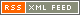### Multi-field alpha attractors

Mar. 12, 2018

The alpha-attractors are a class of inflationary models, that match the current data with the spectral index of the scalar perturbation. The moduli space of the inflaton field is the hyperbolic geometry and the curvature of the moduli space determines the value of the tensor-to-scalar ratio. Some class of 4D N=1 supersymmetric reduction of superstring theory, M-theory, or maximal supergravity are known to have multiple fields with the hyperbolic moduli space geometry. In this seminar, I will describe alpha-attractors with multiple moduli fields and show its applications. In particular, we will focus on the merger of moduli fields, which can enhance the value of the tensor-to-scalar ratio effectively. Also, the supersymmetric version of the alpha-attractors has the superpartner scalar of the inflaton. I will show that the superpartner axion field is a good candidate for cold dark matter.

calendar page